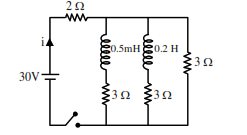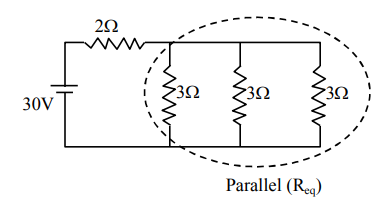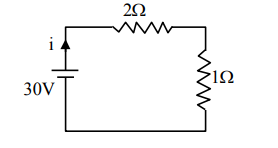# For the given circuit the current i through the battery when the key in closedQuestion:

For the given circuit the current i through the battery when the key in closed and the steady state has been reached is_________.1. 6 A

2. 25 A

3. 10 A

4. 0 A

Correct Option: , 3

Solution:

In steady state, inductor behaves as a conducting wire.

So, equivalent circuit becomes$\frac{1}{\mathrm{R}_{\mathrm{eq}}}=\frac{1}{3}+\frac{1}{3}+\frac{1}{3}=1$

$\Rightarrow R_{\text {eq }}=1 \Omega$

$\Rightarrow$ Circuit becomes$\Rightarrow \mathrm{i}=\frac{30}{3}=10 \mathrm{~A}$